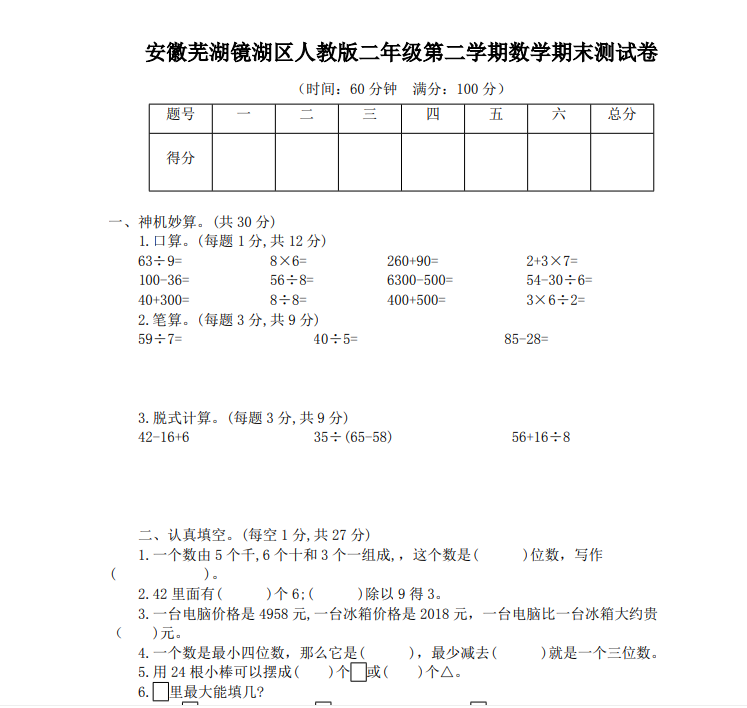1.口算。(每题 1 分,共 12 分)
63÷9= 8×6= 260+90= 2+3×7=
100-36= 56÷8= 6300-500= 54-30÷6=
40+300= 8÷8= 400+500= 3×6÷2=
2.笔算。(每题 3 分,共 9 分)
59÷7= 40÷5= 85-28=评论信息